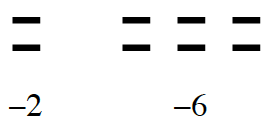Home > CC2MN > Chapter cc23 > Lesson cc23.1.2 > Problem3-19

3-19.

Show the numbers of $+$ and $-$ tiles represented by each expression below. Sketch each model and state the number that it represents. Homework Help ✎

1. $(-2)+(-6)$

If you need help starting the problem, look at the Math Notes box in Lesson 2.2.4.

Begin by sketching a model of the integers.Use the model you just sketched to help you determine what number the expression represents.

This expression represents $-8$.

1. $(-2)+4+(-2)$

Use part (a) as a guide for this problem. Remember to look for and eliminate zeros.

This expression represents $0$. Remember to draw a diagram to model this expression.

1. $5+(-8)$

Use part (a) as a guide for solving this problem.